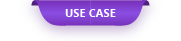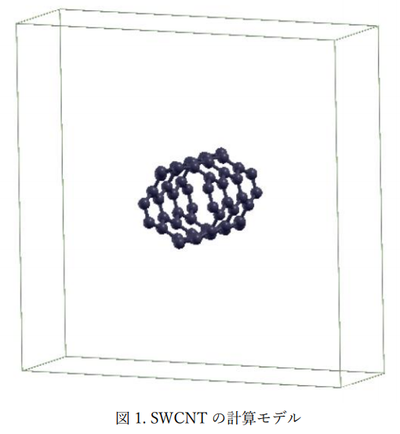The classical molecular dynamics method has been mainly used so far as a method for theoretically evaluating the thermal conductivity of carbon nanotubes.

# Calculation of lattice thermal conductivity of carbon nanotubes using neural network MD

The classical molecular dynamics method has been mainly used so far as a method for theoretically evaluating the thermal conductivity of carbon nanotubes. However, the high thermal conductivity of carbon nanotubes cannot be fully evaluated by the conventional classical force field. On the other hand, high-precision calculations using first-principles calculations can only handle small-scale models, so calculation costs are high when trying to calculate a wide variety of structures for carbon nanotubes. Therefore, the lattice thermal conductivity of single-walled carbon nanotubes (SWCNTs) is evaluated using the neural network MD (NNMD), which is faster than the first-principles calculation and more accurate than the existing molecular dynamics calculations, and the first-principles calculation is performed. And compared with the result of the conventional classical force field.

### 1. Computational model

① NNMD teacher data creation

For the teacher data for creating the potential force field used for NNMD, we used about 3000 structural models in which random atomic displacements were given to SWCNTs with different diameter sizes.

② Phonon calculation model

The SWCNT model used in the calculation used a supercell with a chirality of (4,4) and a unit cell expanded to 1 × 1 × 3 for phonon calculation (see Fig. 1). In addition, the internal structure of the unit cell was optimized by the calculation method used.

### 2. Calculation condition

Advance / NeuralMD was used to create the interatomic potential used for NNMD, and the teacher data used to learn the potential was calculated using first-principles calculations. In addition, the phonon characteristics were calculated using the real-space displacement method, and the lattice thermal conductivity was evaluated by non-harmonic lattice dynamics. This time, the first-principles calculation and the classical force field calculation are used for the calculation of the atomic force used for the phonon calculation, and the NNMD and the conventional force field (Tersoff) are compared as the classical force field. Quantum ESPRESSO is used for all first-principles calculations, and LAMMPS is used for classical force field calculations. The phonon calculation code ALAMODE  was used for the phonon calculation. The details of the calculation conditions are shown in Table 1.

### 3. Calculation result

① Phonon dispersion

Figure 2 shows the phonon dispersion curves obtained from each calculation method. In Tersoff, which is a conventional classical force field, a negative frequency appears, which is a result that deviates greatly from the result of first-principles calculation. On the other hand, it can be seen that the calculation result of the phonon variance of NNMD is close to the result of the first-principles calculation.

② Lattice thermal conductivity

Figure 3 shows the lattice thermal conductivity obtained from each calculation method. The conventional classical force field, Tersoff, is an order of magnitude smaller than the first-principles calculation result, but the lattice thermal conductivity of NNMD is close to the first-principles calculation result.

From the above, it was confirmed that the calculation result of the phonon thermal characteristics of NNMD is close to the first-principles calculation result, and the accuracy is higher than that of the conventional classical force field.

### 4. Calculation time

Table 2 shows the calculation time required for this calculation. It can be seen that NNMD can be calculated about 10 times faster than first-principles calculation.

### 5. References

 T. Tadano, Y. Gohda, and S. Tsuneyuki, J. Phys .: Condens. Matter 26, 225402 (2014)

Original Source from: https://ctc-mi-solution.com/ニューラルネットワークmdを用いたカーボンナノチ/